# Factoring PolynomialsPage 1

#### WATCH ALL SLIDES

Slide 1## Factoring Polynomials

Slide 2A “Difference of Squares” is a binomial (*2 terms only*) and it factors like this:

Slide 3Factoring a polynomial means expressing it as a product of other polynomials.

Slide 4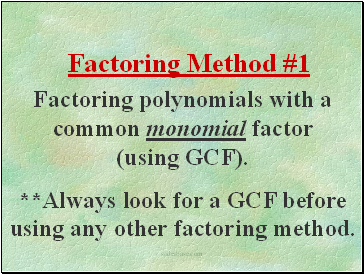Factoring polynomials with a common monomial factor (using GCF).

**Always look for a GCF before using any other factoring method.

Factoring Method #1

Slide 5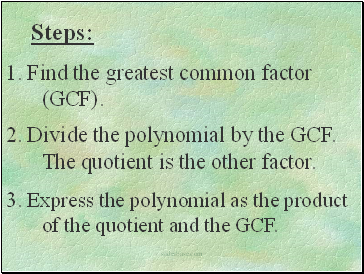Steps:

1. Find the greatest common factor (GCF).

2. Divide the polynomial by the GCF. The quotient is the other factor.

3. Express the polynomial as the product of the quotient and the GCF.

Slide 6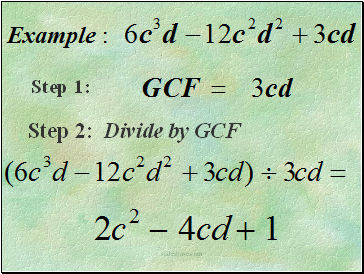Step 1:

Step 2: Divide by GCF

Slide 7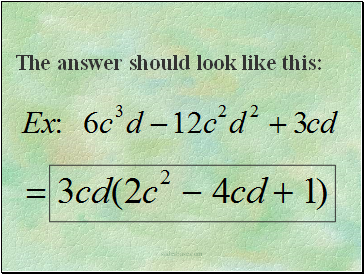The answer should look like this:

Slide 8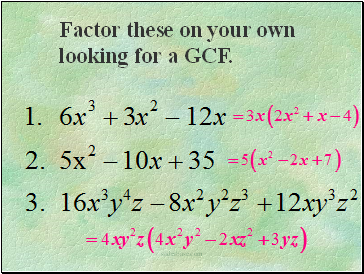Factor these on your own looking for a GCF.

Slide 9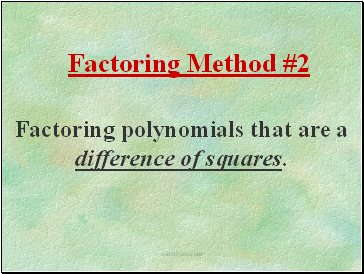Factoring polynomials that are a difference of squares.

Factoring Method #2

Slide 10Slide 11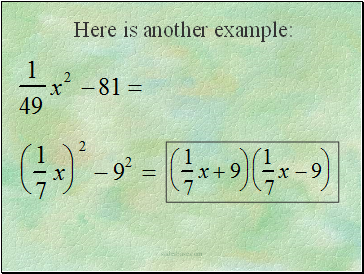Here is another example:

Slide 12Slide 13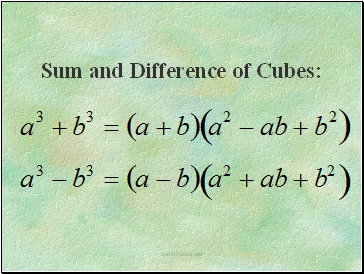Sum and Difference of Cubes:

Slide 14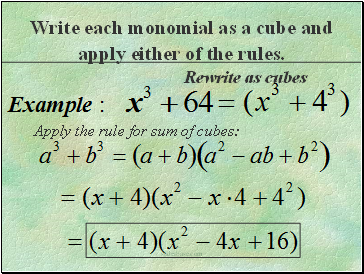Rewrite as cubes

Write each monomial as a cube and apply either of the rules.

Slide 15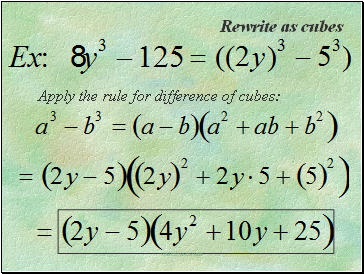Rewrite as cubes

Slide 16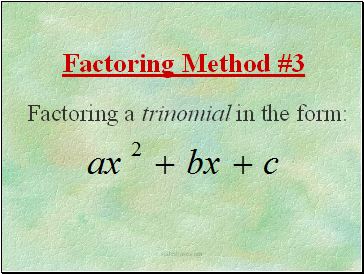Factoring Method #3

Slide 17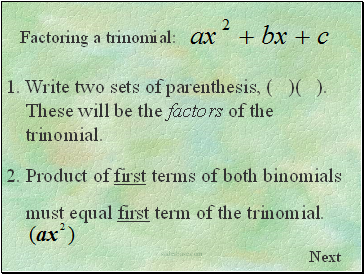Next

2. Product of first terms of both binomials

must equal first term of the trinomial.

1. Write two sets of parenthesis, ( )( ). These will be the factors of the trinomial.

Slide 18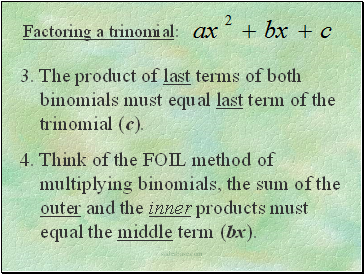Go to page:
1  2  3